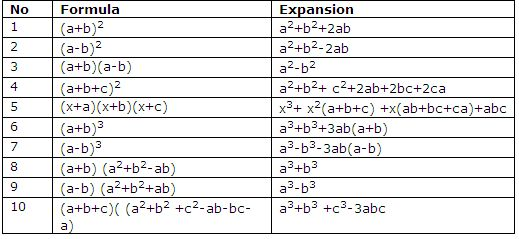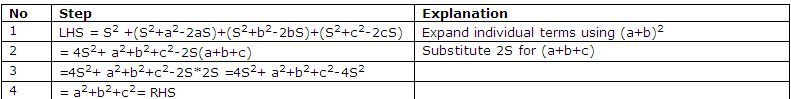Name: ___________________Date:___________________

 Email us to get an instant 20% discount on highly effective K-12 Math & English kwizNET Programs!

High School Mathematics - 25.1 Conditional IdentitiesExample: If a+b+c = 2S prove that S2+(S-a)2+ (S-b)2+(S-c)2= a2+b2+c2Directions: Prove the following.
 Q 1: Answer: Q 2: If a+b+c = 0 prove that a2/bc+ b2/ca+ c2/ab = 3 Answer: Q 3: Answer: Q 4: If a+b+c = 2S prove that a2+b2- c2+2ab/ a2-b2+ c2+2ac = (S-c)/(S-b) Answer: Q 5: If a+b+c 0 and a3+b3+c3 =3abc prove that a=b=c Answer: Q 6: Answer: Q 7: If a+b+c = 0, prove that a3+b3+c3 = 3abcAnswer: Q 8: Prove that (x+y)(x+z) = x(x+z)+y(x+z)= x2+xz+xy+yz= x2+x(y+z)+yzAnswer: Question 9: This question is available to subscribers only! Question 10: This question is available to subscribers only!